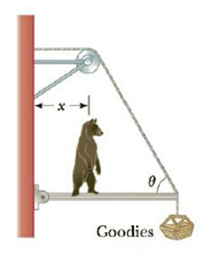Chapter 8, Problem 28P

Chapter
Section
Textbook Problem

A hungry bear weighing 700. N walks out on a beam in an attempt to retrieve a basket of goodies hanging at the end of the beam (Fig. P8.28). The beam is uniform, weighs 200. N, and is 6.00 m long, and it is supported by a wire at an angle of θ = 60.0°. The basket weighs 80.0 N. (a) Draw a force diagram for the beam, (b) When the bear is at x = 1.00 m, find the tension in the wire supporting the beam and the components of the force exerted by the wall on the left end of the beam, (c) If the wire can withstand a maximum tension of 900. N, what is the maximum distance the bear can walk before the wire breaks?Figure P8.28

(a)

To determine
Draw a force diagram for the beam.

Explanation

The Free body diagram for force is given below

(b)

To determine
The tension of the wire supporting the beam and the components of the force exerted by the wall on the left end of the beam.

(c)

To determine
The maximum distance the bear can walk before the wire breaks xmax

Still sussing out bartleby?

Check out a sample textbook solution.

See a sample solution

The Solution to Your Study Problems

Bartleby provides explanations to thousands of textbook problems written by our experts, many with advanced degrees!

Get Started

The body's adipose tissue has a virtually infinite capacity to store fats. T F

Nutrition: Concepts and Controversies - Standalone book (MindTap Course List)

What does the word apparent mean in apparent visual magnitude?

Horizons: Exploring the Universe (MindTap Course List)

What is hereditarianism, and what is the invalid assumption it makes?

Human Heredity: Principles and Issues (MindTap Course List)

Match the term listed in Column A with its definition from Column B

Nutrition Through the Life Cycle (MindTap Course List)